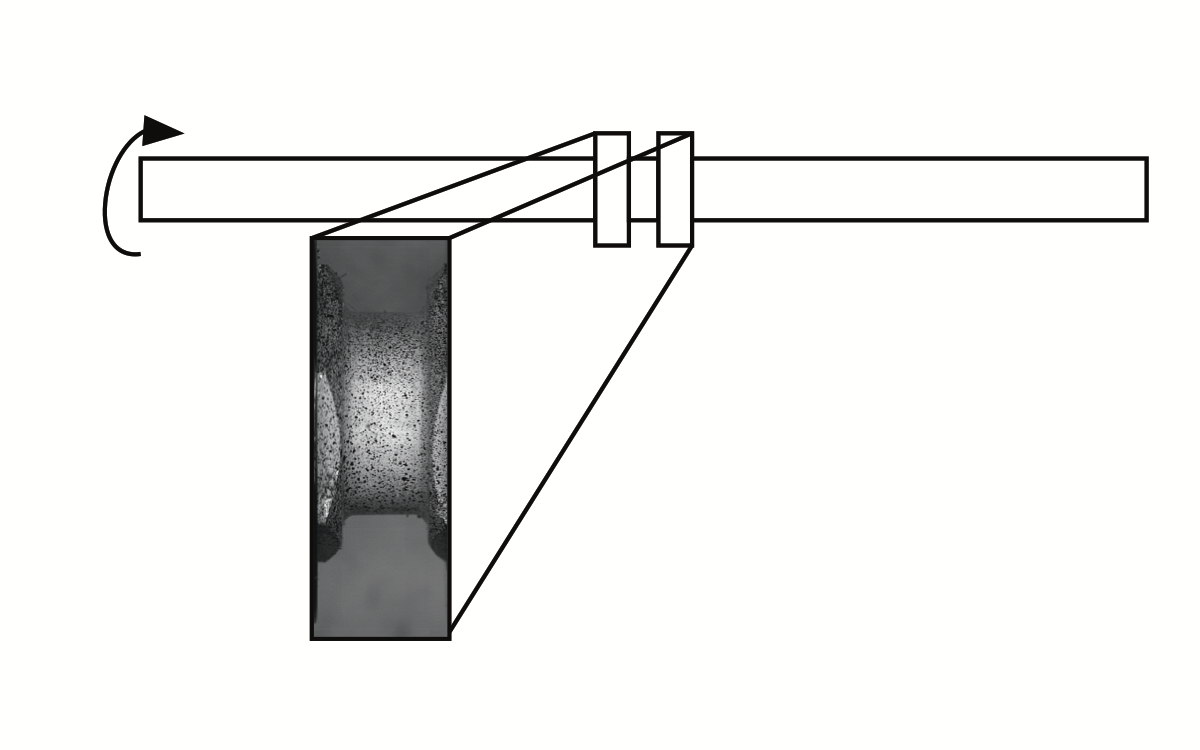# Solver stability issues in DIC.jl

Brent Halonen
June 26th, 2019

I have been working on a functional Digital Image Correlation program in the Julia language. The approach seeks to find 2 mathematical functions (in particular polynomials) to approximate the displacement field. (One of the functions predicts x displacement, the other y displacement). The idea is that the displacement is very simple in the space-time domain, and could be represented by a small set of polynomial parameters. Anything that isn't simple (like a tear) would not be represented well in this domain. One feature is that strain rate heat maps will be extremely easy to create, which is of interest in the high strain rate testing domain. The data I am working with is that of a twisting motion to produce a torsional stress on a tube. Force is imparted as displayed below.Some of the time this idea works fairly well. Here the displacement field is closely converged to the actual displacement that you would expect.The algorithm works as follows: a test set of local neighborhoods is constructed using Sobol sampling in the region of interest, with neighborhoods iteratively assigned to frames. A cost function for the polynomial parameters is constructed as follows:

1. Combine parameters and model to form prediction polynomial
2. Iterate through local area samples
3. Predict a location in a projected image for each polynomial
4. Measure the distance between the values of the original image and the predicted results, where bicubic interpolation is used to determine predicted pixel values

Then, a numerical optimization is run on the polynomial parameters to minimize the constructed cost function. Since a gradient cannot be established easily because of the discrete video samples, we selected the Nelder-Mead approach.

There are some convergence issues with the selected approach. Often, the algorithm will go offroading, probably due to a flat error function outside of a local neighborhood. Here is an example of what the error function might look like:This can create some interesting "art," as the polynomial coefficients end up predicting totally crazy results. Clearly, some fine-tuning is needed.